# Custom Inductor (B-H Curve)

This example shows a comparison in behavior of a linear and nonlinear inductor. Starting with fundamental parameter values, the parameters for linear and nonlinear representations are derived. These parameters are then used in a Simscape™ model and the simulation outputs compared.

### Open Model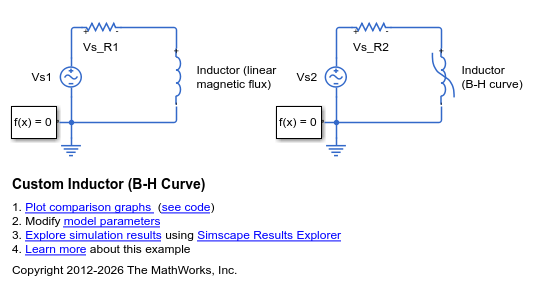### Specification of Parameters

Fundamental parameter values used as the basis for subsequent calculations:

• Permeability of free space,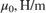• Relative permeability of core,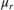• Number of winding turns,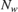• Effective magnetic core length,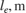• Effective magnetic core cross-sectional area,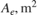• Core saturation begins,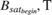• Core fully saturated,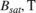### Calculate Magnetic Flux Density and Magnetic Field Strength Data

Where:

• Magnetic flux density,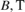• Magnetic field strength,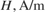Linear representation:

•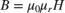Nonlinear representation (including coefficient, a):

•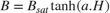### Display Magnetic Flux Density Versus Magnetic Field Strength

The linear and nonlinear representations can be overlaid.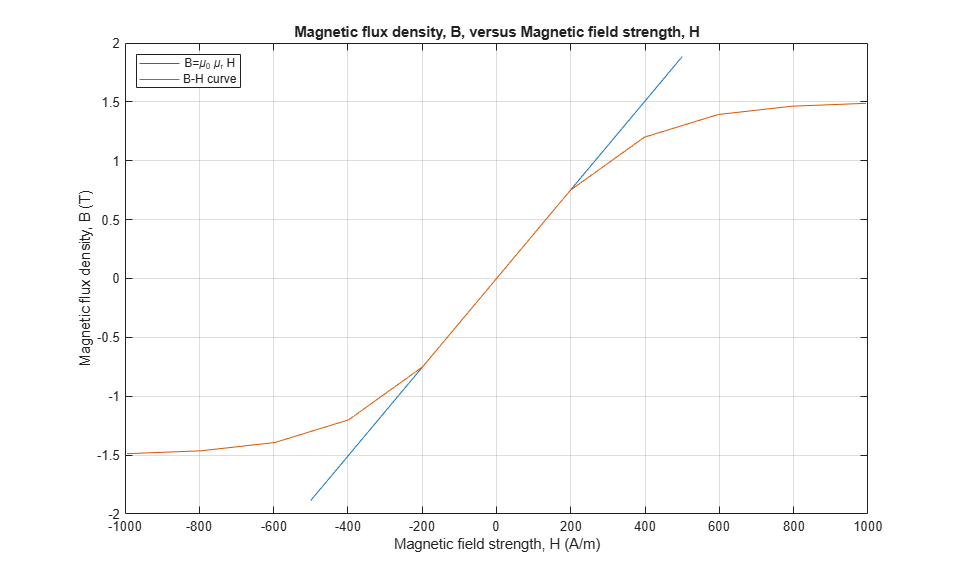### Calculate Magnetic Flux and Current Data

Where:

• Magnetic flux,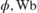• Current,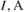Linear representation:

•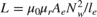•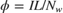Nonlinear representation:

•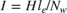•### Display Magnetic Flux Versus Current

The linear and nonlinear representations can be overlaid.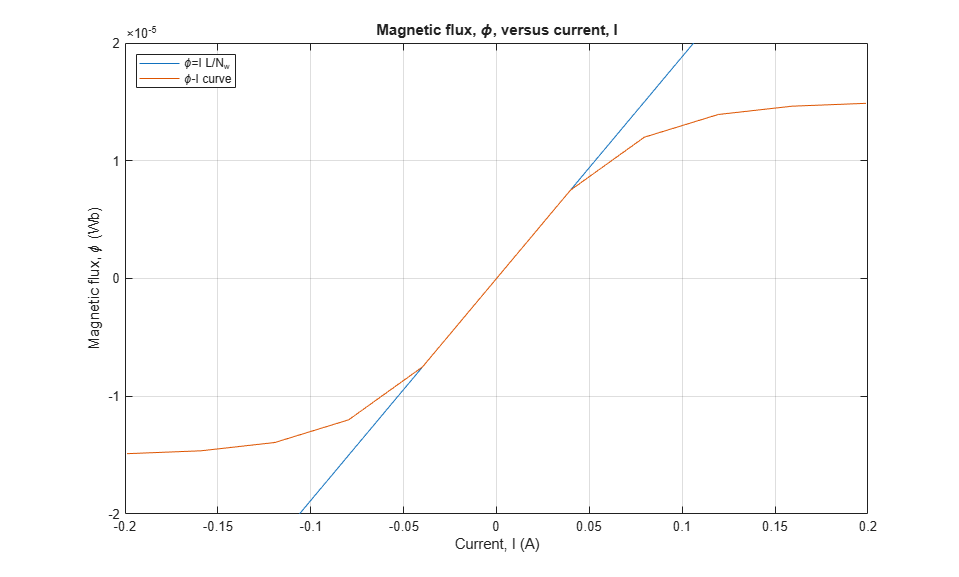### Use Parameters in Simscape Model

The parameters calculated can now be used in a Simscape model. Once simulated, the model is set to create a Simscape logging variable, simlog_CustomInductorBHCurve.

### Conclusion

The state variable for both representations is magnetic flux,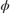. Current, I, and magnetic flux,, can be obtained from the Simscape logging variable, simlog_CustomInductorBHCurve, for each representation. Overlaying the simulation results from the representations permits direct comparison.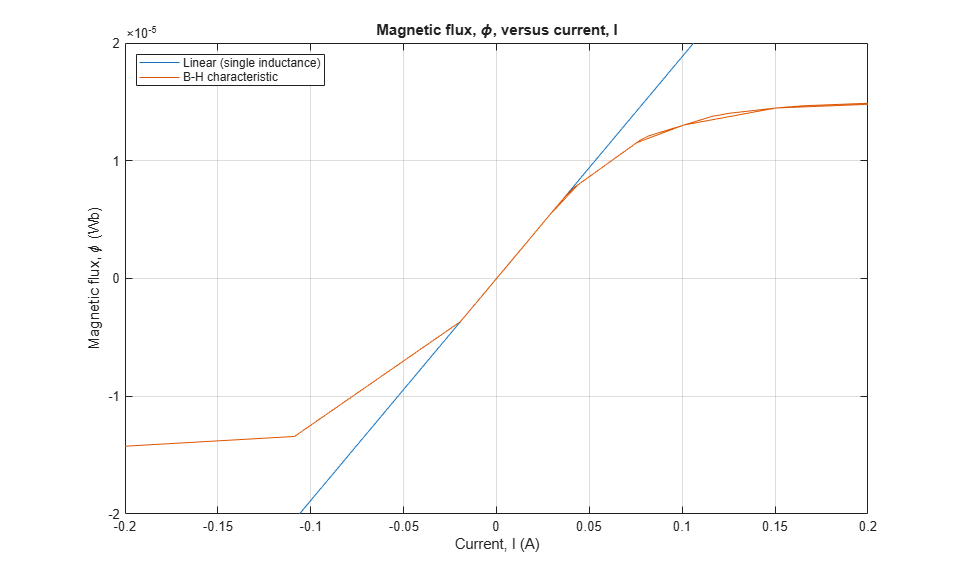### Results from Real-Time Simulation

This example has been tested on a Speedgoat Performance real-time target machine with an Intel® 3.5 GHz i7 multi-core CPU. This model can run in real time with a step size of 50 microseconds.